### The Multiplicative Hazards Model

Consider a set of n subjects such that the counting process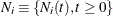for the ith subject represents the number of observed events experienced over time t. The sample paths of the process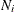are step functions with jumps of size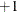, with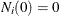. Letdenote the vector of unknown regression coefficients. The multiplicative hazards function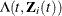foris given by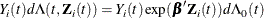where

•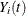indicates whether the ith subject is at risk at time t (specifically,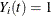if at risk and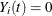otherwise)

•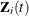is the vector of explanatory variables for the ith subject at time t

•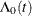is an unspecified baseline hazard function

See Fleming and Harrington (1991) and Andersen et al. (1992). The Cox model is a special case of this multiplicative hazards model, whereuntil the first event or censoring, andthereafter.

The partial likelihood for n independent triplets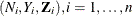, has the form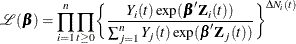where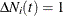if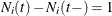, and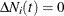otherwise.